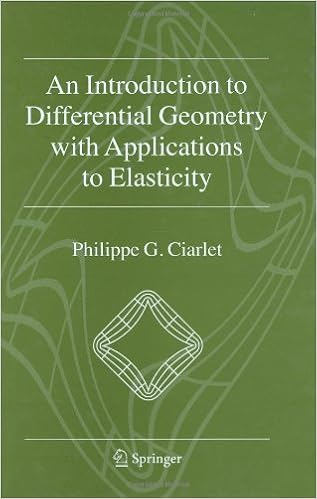## Monday, September 21, 2015

### An Introduction to Differential Geometry with Applications to Elasticity by Philippe G. CiarletThis monograph presents the basic theorems of differential geometry in three-dimensional space, including a thorough coverage of surface theory. By means of a series of carefully selected and representative mathematical models this monograph also explains at length how these theorems are used in three-dimensional elasticity and in shell theory. The presentation is essentially selfcontained, with a great emphasis on pedagogy. In particular, no 'a priori' knowledge of differential geometry or of elasticity theory is assumed, the only requirements are a reasonable knowledge of basic analysis, functional analysis, and some acquaintance with ordinary and partial differential equations.